HPS 0410 Einstein for Everyone

Back to main course page

# Einstein's Pathway to General Relativity

John D. Norton
Department of History and Philosophy of Science
University of Pittsburgh

Linked document: Uniform Acceleration in a Minkowski Spacetime

We have followed a simple pathway to the main ideas of the general theory of relativity. We started with the geometrical notion of the curvature of space and saw how that geometrical notion can be extended from space to spacetime. We then found the resulting theory of curved spacetime not just to cover a curved geometry of space, but gravitational phenomena as well.

This pathway to the theory was not Einstein's. His was more indirect, more inspired, more tortured and more fallible. The final theory emerged after Einstein struggled for seven years with many things: strong hunches about what the theory should say physically, vivid thought experiments to support the hunches, lengthy explorations into new mathematics, errors and confusions that thoroughly derailed him and a final insight that rescued him from exhaustion and desperation.

 1907 1908   1910   1911   1912   1912-1913   1913   1914   1915 The seven years of work divides loosely into two phases. The earlier phase of his work from 1907 to 1912 was governed by powerful physical intuitions that seemed as much rationally as instinctively based. Einstein felt a compelling need to generalize the principle of relativity from inertial motion to accelerated motion. He was transfixed by the ability of acceleration to mimic gravity and by the idea that inertia is a gravitational effect. These ideas finally issued in a theory of static gravitational fields in 1912. In it, gravity bends light and slows clocks and the speed of light varies from place to place. The major transition to general relativity came after the summer of 1912 and into early 1913. Einstein struggled to incorporate these ideas into a more general physical theory. He was drawn to use the mathematics of curvature as a means of formulating the new theory. To learn the mathematics, he collaborated with his mathematician friend, Marcel Grossmann. Together, they produced the first draft of the general theory of relativity. The remaining years, 1913 to 1915, were devoted to the labored work of correcting and perfecting his draft of 1913. As the mathematics of curvature took a more controlling position in the later phases, Einstein's work began to change. The theorizing was governed increasingly by notions of mathematical simplicity and naturalness. When the theory was completed, Einstein's starting point was quite distant.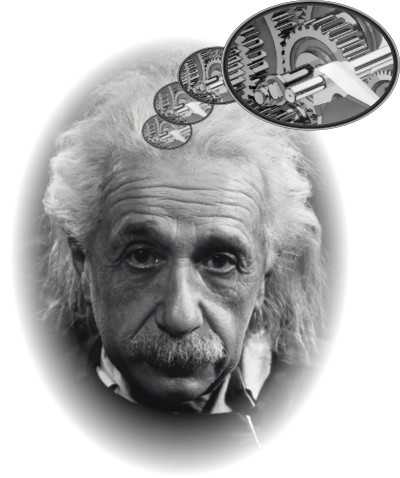It is impractical in this chapter to review all these considerations. Einstein's intricate mathematical struggles in the later years cannot easily be described in informal terms. However some of his earlier physical reflections are so famous and so characteristic of Einstein, that they must be mentioned. Finally a caution to those seeking to learn general relativity from these pages. The considerations below are the ones that guided Einstein towards general relativity. Whether these guides persist as founding principles of general relativity remains debated today. In particular, there is increasing consensus that the final theory does not extend the principle of relativity to accelerating motion in any interesting way. For an elaboration, see the later chapter, The Relativity of Accelerated Motion. You should treat what follows as interesting reports on Einstein's intellectual biography. You may well find it hard to connect some of the ideas to be laid out below with the final theory.

### The Starting Point

Einstein's first concrete steps on his pathway to general relativity came in 1907 when he was commissioned by Johannes Stark to write a review article on relativity theory for Stark's journal Jahrbuch der Radioaktivitaet und Electronik. The exercise was, apparently, quite straightforward. In his 1905 theory, Einstein had offered a new account of space and time. Since the theories of physics were all set in space and time, physicists needed to be assured that these theories could be maintained; or, if not, shown how they should be adjusted to fit with Einstein's new theory.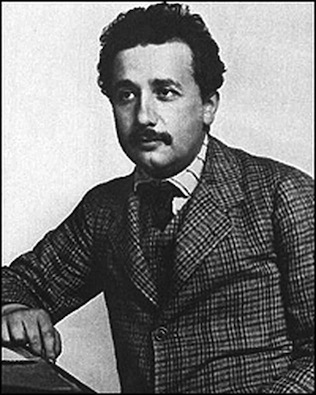The exercise proceeded well. Electrodynamics actually needed no adjustment. Einstein's 1905 theory of relativity had been created to fit with the existing theory. The mechanics of bodies required adjustments to the notions of energy, momentum and mass. The most prominent of these was the famous equivalence E=mc2. Einstein also sketched a relativistic treatment of thermodynamics, the theory of heat and work.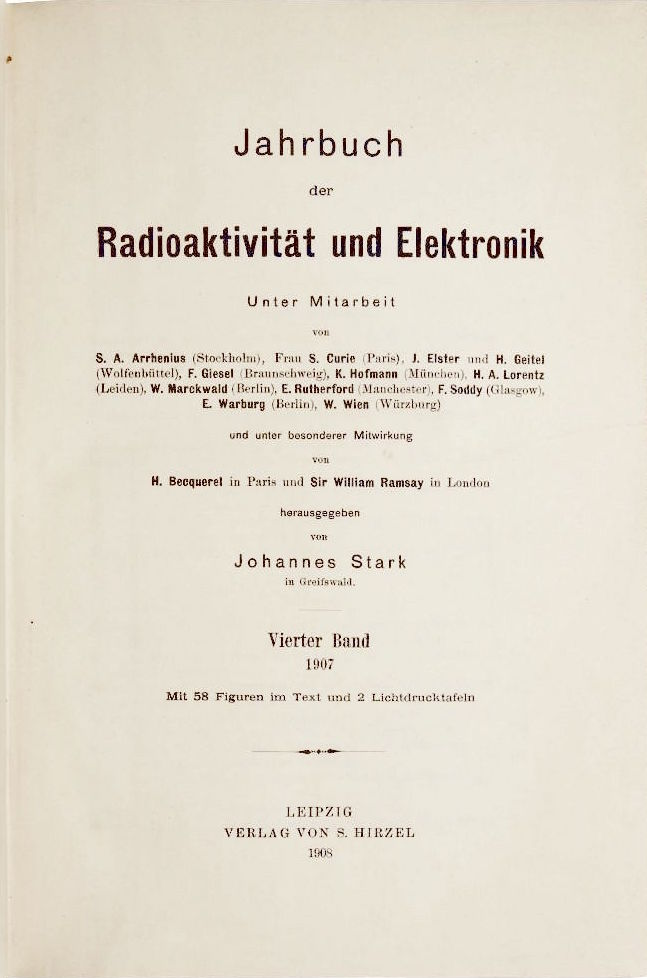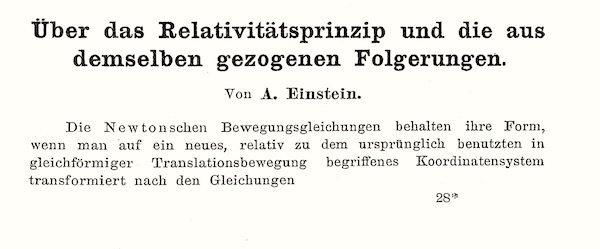Then came gravity. Newton's celebrated theory of gravitation presumed instantaneous action at a distance. The sun now exerts a gravitational force on the earth now with a magnitude set by Newton's inverse square law. The key part was the "now." If the sun were to move slightly, the resulting alteration in the force it exerts on the earth would be felt by us instantaneously according to Newtonian theory.That means that Newton's theory depends upon a notion of absolute simultaneity. A change there is felt here at the same moment. However Einstein's 1905 theory had banished absolute simultaneity from physics. Different observers would judge different pairs of spatially separated events to be simultaneous. Newton's theory had to be adjusted to accommodate this new relativity.

## Adjusting Newton's Theory of Gravitation

The change needed was, apparently, straightforward. In the revised theory, a change in the sun should not be felt here on earth instantly, but only after a time lag of around 8 1/3 minutes, the approximate time light takes to propagate from the sun to the earth. Then absolute simultaneity would no longer be needed in the theory.

This meant that Newton's theory needed to be adjusted to look more like electrodynamics. In the latter theory, effects do not propagate instantly in the electromagnetic field; they propagate in waves at the speed of light. There were many ways to make the adjustments Newton's theory needed. All of them produced very small changes in the predictions of the theory. While one might not be sure precisely which of the many adjustments was the right one to pick, there did not seem to be any major problem. Rather the issue was a surfeit of good solutions. Or so believed other leading thinkers of Einstein's time, such as the great French mathematician, Henri Poincaré, and the inventor of spacetime, Hermann Minkowski.

Einstein, however, did not see it that way. He examined gravitation theories, modified to allow for a finite time of propagation of effects, and found a result that aroused great suspicions in him. In the modified theories, the distance fallen by a body varies according to its sideways motion. In the simplest case, the body would fall a shorter distance if it has some sideways velocity.The differences in the distances fallen were very small and not likely to be detectable in an experiment. Nonetheless they bothered Einstein. They contradicted the exact correctness of Galileo's old observation that all bodies fall alike, even though the differences were far too small to be detectable by the methods available to Galileo.

Other physicists of the time were aware of this effect, but discounted it as too small to be of any concern. Einstein did not. It meant that the way a body fell would depend on the energy of the body. We can only guess now why that bothered Einstein so much. It might be that Einstein imagined that a hot body, consisting of many small atoms in thermal motion, might fall differently from a cold one according to these theories.

Einstein was still a clerk in the Bern patent office in 1907. Yet he came to the extraordinary conclusion that an adequate theory of gravitation could not be devised within the confines of his existing theory of relativity.

## "The Happiest Thought of My Life"

It was while pondering this problem that Einstein hit upon what he later described as "the happiest thought of my life." If began when he suddenly saw new significance in a commonplace of Newtonian gravity. A body in free fall in Newtonian gravity does not feel its own weight. This effect is very familiar to us now. We have all watched space-walkers floating weightlessly outside their capsules. They are in free fall above the earth, orbiting with their space stations, and that free fall cancels their weight.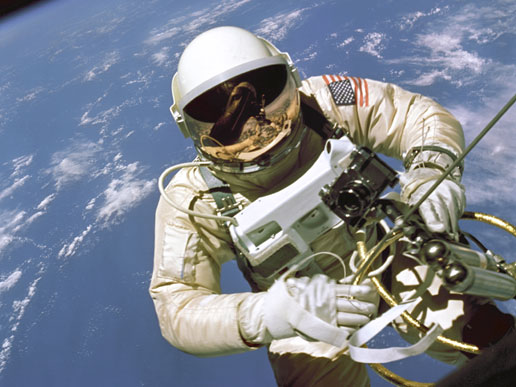This effect came about from an apparently accidental agreement of two quantities in Newtonian theory: the inertial mass of a body happens to equal its gravitational mass exactly. Einstein now believed that this equality could be no accident. He needed to find a gravitation theory in which this equality is a necessity. The inertial mass of a body measures its resistance to acceleration when a force is applied to it. The gravitation mass of body measures how it responds to a gravitational field. For more, see this.

The immediate outcome of this reflection was Einstein's "principle of equivalence." It formed the basis of the concluding Part V of his 1907 Jahrbuch article. There he suggested that gravitation required an extension of special relativity based on the principle of equivalence.

## The Principle of Equivalence

There are very many formulations of the principle of equivalence in the literature. Most of them pick up directly on the idea of weightlessness in free fall. They assert that free fall transforms away a gravitational field in some tiny volume of space. While this is a common formulation of the principle in text books, it is troubled. Free fall transforms away gross effects of gravitation. But, in Einstein's final theory, it does not transform away the effects of spacetime curvature. In that sense, free fall does not transform away gravity in the final theory.

For an elaboration on these foundational issues, see The Principle of Equivalence in the later chapter "The Philosophical Significance of General Relativity: The Relativity of Accelerated Motion."

 Einstein later complained about this version of the principle, objecting that one could not in general transform away an arbitrary gravitational field over an extended region of space. His original formulation and the one to which he adhered for his entire life proceeded differently. He turned around the original idea of free fall eradicating gravitation. Acceleration can also produce a gravitational field. For more, see John D. Norton,"What was Einstein's Principle of Equivalence?" Studies in History and Philosophy of Science, 16 (1985) , pp. 203-246; reprinted in D. Howard and J. Stachel (eds.), Einstein and the History of General Relativity: Einstein Studies Vol. I, Boston: Birkhauser, 1989, pp.5-47.

More specifically, Einstein took the case of special relativity without gravitation. He now imagined a uniformly accelerated observer, in relation to whom all free objects would accelerate. That state of space found by the observer, Einstein asserted in his principle of equivalence, is a homogeneous gravitational field. In this case, uniform acceleration and homogeneous gravitation are equivalent.

Einstein developed the idea in one of his best known thought experiments. He asked us to imagine a physicist who awakens in a box. Unknown to the physicist, the box is in a distant part of the space of special relativity and is being accelerated uniformly in one direction by the tug of some agent. If the physicist were to release objects in the box, they would be left behind by the accelerating box; they would move inertially, while the box accelerated. This figure shows this for two bodies of different mass at rest and a third body that has a horizontal inertial motion.The physicist inside the box would find that the released masses to accelerate in a direction opposite to the box's acceleration. The physicist would judge there to be a field inside the box pulling on all free bodies.Now comes the key point. All bodies released by the physicist would fall exactly alike, no matter what their mass or composition. So the field found by the physicist inside the box would manifest the signature property of a gravitational field: it would accelerate all bodies exactly alike.

One might be tempted to say that the field inside the box is just an "inertial field," some sort of fake gravitational field. Einstein's assertion was otherwise. The field created by motion in the box just is a full-blown, authentic homogeneous gravitational field.

Principle of Equivalence
The inertial effects inside a uniformly accelerated box in gravitation free space are equivalent to those of a homogeneous gravitational field; more tersely, uniform acceleration creates a homogeneous gravitational field.

The equivalence just asserted may seem benign. It seems just to codify an equivalence in the way bodies fall in two cases. In fact the assertion is strong, for it asserts that the equivalence applies to all processes, not just to the fall the bodies. That means that it applies also to all processes involving fields, such as electric and magnetic fields.

You will see why Einstein found this principle attractive. His efforts to produce a relativistic theory of gravity had failed since he could find no theory in which all bodies fell alike, no matter what their mass or composition. The gravitational field delivered by the principle of equivalence was assured to have this property. In particular, the sideways motion of a body would have no effect on its rate of fall. The field generated in this thought experiment did not have the defect of the earlier theories.

## Relativity of Inertia ("Mach's Principle")

What also attracted Einstein in this analysis was that it promised to remedy a defect he perceived in both Newton's physics and in special relativity. In both, you will recall, it is just a brute fact that certain motions are distinguished as inertial. This, in Einstein's view, was worrisome. It was no better than the original idea that there is an ether state of absolute rest. There seemed to Einstein no good reason for why one state should be the absolute rest state rather than another. Correspondingly, Einstein saw no good reason for why some motions should be singled out as inertial and others as accelerating.

In 1916, Einstein formulated this worry in a thought experiment. He imagined two fluid bodies in a distant part of space. These bodies, the reader quickly infers, are like stars or planets, which form roughly spherical shapes under their own gravity. Einstein further imagined that there is relative rotation between the two bodies about the axis that joins them. This relative rotation is verifiable by observers on each body, who can trace out the motion of the other body. Each would judge the other to be rotating.It can happen in ordinary Newtonian physics that one of these bodies is not rotating with respect to an inertial frame and the other one is. In that case, the second rotating body will bulge but not the first. This effect arises on the earth. It rotates about the axis of its north and south poles. It bulges slightly at the equator as a result of centrifugal forces that seek to fling the matter of the earth away from this axis.

It would be entirely unacceptable, Einstein now asserted, were this to happen to two spheres in an otherwise empty space. For there is no difference in the observable relations between the two spheres. Each rotates with respect to the other. So why should just one bulge? The supposition of Newton's absolute space or of inertial systems, Einstein protested, was an inadequate explanation. Einstein demanded something observable to make the difference.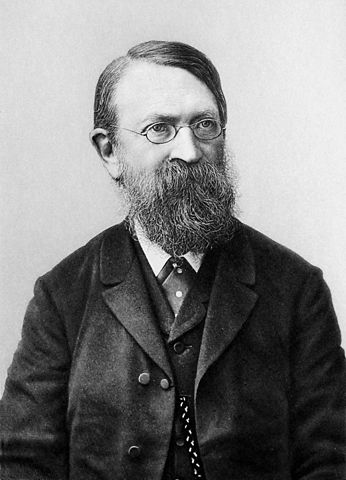Einstein was an avid reader of the physicist-philosopher Ernst Mach. In Mach's writings, Einstein had found what seemed to be a solution to the problem. Mach seemed to be proposing, Einstein thought, that the privileging of certain states of motion is due to the distribution of matter in the universe. Why is our frame of reference inertial? It is because the stars are at rest in our frame. Why is my wording so careful here? it is not clear that what Einstein reported Mach as saying is what Mach actually said. For more, see John D. Norton, "Mach's Principle before Einstein." in J. Barbour and H. Pfister, eds., Mach's Principle: From Newton's Bucket to Quantum Gravity: Einstein Studies, Vol. 6. Boston: Birkhäuser, 1995, pp.9-57. Download.

When we try to accelerate, we feel inertial forces. These are the forces that make us dizzy when we spin in a fun fair; or they are the forces that throw our coffee in the air when our airplane hits an air pocket.

These forces, Einstein understood Mach to assert, arise from an interaction between the mass of our body (and our coffee) and all the other masses of the universe, distributed in the stars. Einstein first called this idea the "relativity of inertia" and later, in 1918, "Mach's Principle."

In the case of Einstein's two fluid spheres, the bulge of one of them would now be explained by the fact that this bulging sphere was rotating with respect to all the other masses of the universe, whereas the other sphere was not. That would be the observable difference between the two fluid bodies.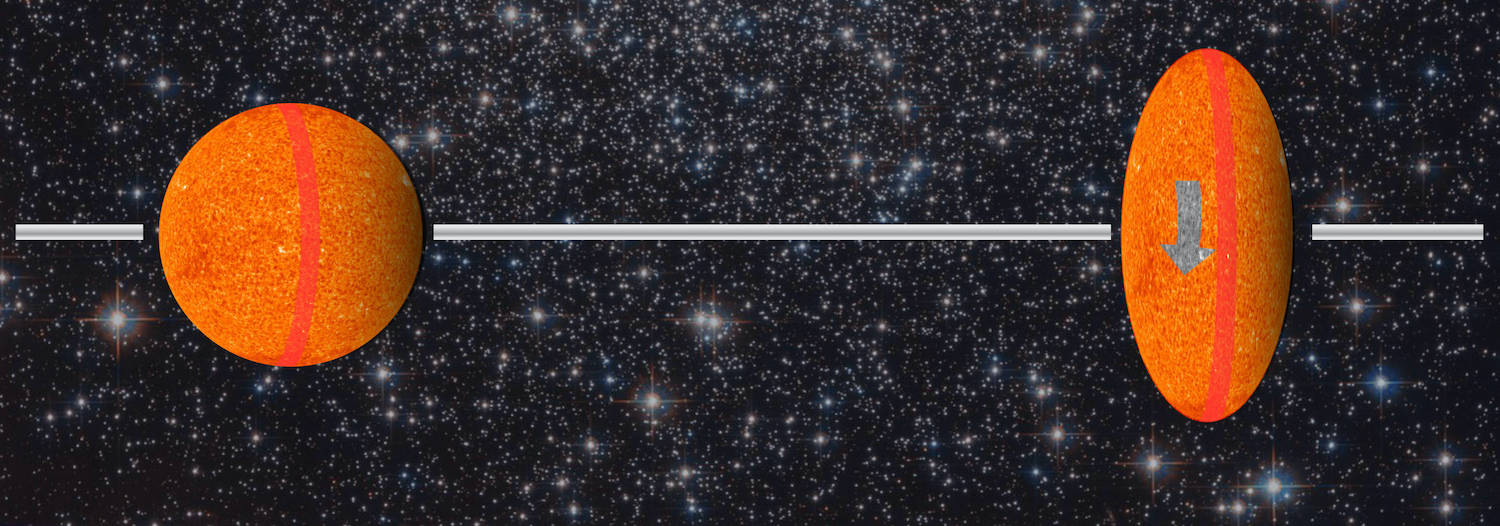Image sources: https://www.nasa.gov/sites/default/files/images/283003main_solar_full.jpg https://www.nasa.gov/sites/default/files/thumbnails/image/hubble_friday_08052016.jpg

This analysis was clearly inspired by Mach's famous account of Newton's bucket experiment. Newton had noted that water in a spinning bucket adopts a concave surface. The concavity is a result, Newton urged, of its rotation with respect to absolute space. No, Mach had responded several hundred years later, all one has in the case of Newton's bucket in rotation with respect to the stars. We cannot know anything more than what our direct observations tell us. All they tell us is that these inertial forces arise when we accelerate relative to the stars.

The weakness of this analysis is that there is no account of how rotation with respect to distant masses could produce these inertial forces. In 1907, Einstein hoped that his emerging theory of gravity would provide the mechanism. It could then satisfy Mach's Principle and, through it, generalize the principle of relativity to acceleration. For in a theory that satisfies Mach's Principle, no state of motion is intrinsically inertial or accelerating. When we see something accelerating, it is not accelerating absolutely in such a theory; it is merely accelerating with respect to the stars. Preferred inertial motions need not enter into the account any more. All motion, accelerated or inertial, would be relative.

To deliver this sort of account of inertial forces, Einstein's theory would need to break down the strict division between inertial and accelerated motion of his special theory of relativity. The principle of equivalence promised to weaken this division. According to it, whether the physicist in the box was to be judged accelerating or not depended on your point of view. An inertial observer would judge the physicist to be accelerating uniformly in a gravitation free space. The physicist would judge him or herself to be unaccelerated in a gravitational field. It was a first step towards generalizing the principle of relativity to acceleration, Einstein believed.

By his own later judgment, Einstein did not, in the end, find a theory that fully satisfied Mach's Principle. The immediate benefit of his new principle of equivalence, however, was that it let Einstein learn a lot about gravitation. For the principle delivered to Einstein one special case of a gravitational field that, he believed, conformed with relativity theory and in which all bodies truly fell alike. Einstein's program of research on gravity in the five years following 1907 was simply to examine the properties of this one special case and to try to generalize them to recover a full theory. His early hope was that the generalization of the principle of relativity would somehow emerge in the course of those investigations.

### Gravitational Slowing of Clocks

Two properties of this special case of the gravitational field were noteworthy. First, Einstein recognized that clocks run at different rates in the box of his thought experiment according to their location. A clock placed lower in the created field runs slower.Einstein immediately generalized that effect to all gravitational fields. Clocks deeper in a gravitational field run slower. A clock in the sun would run slower than one on earth--if only we could have a clock in the sun without it being destroyed by the heat of the sun. It turns out we can find clocks in the sun. Radiating atoms radiate in very definite frequencies of light according to which element they are. That means that they behave like little clocks. Their running slower is manifested in a slight reddening of the light they emit. Einstein computed an effect on the wavelength of sunlight of one part in two million.

While Einstein did not use spacetime diagrams in 1907, they provide an easy way to see that clocks run at different rates according to their position when they accelerate in a Minkowski spacetime. The effect is driven almost entirely by the relativity of simultaneity.

 The spacetime diagram shows two clocks A and B accelerating together towards the right in a Minkowski spacetime. The numbers show the proper time elapsed along each clock's worldline and thus the time each clock reads. The hypersurfaces of simultaneity are those of the inertial observer on the left of the figure. According to that inertial observer, the two clocks run at the same speed, at least for the initial portion of their acceleration. Why don't the two clocks run at exactly the same speed? This is an artifact of how uniform acceleration arises in a Minkowski spacetime. Observers on the clocks judge the distance between them to stay the same. Therefore an inertial observer will judge this distance to contract. As a result, the inertial observer will judge the two clocks to accelerate at slightly different rates; the difference will be just enough to give the length contraction effect. This means that, in the same time, the A clock will achieve a greater speed than the B clock, according to the inertial observer's judgments of simultaneity. Hence the inertial observer will judge the A clock's reading to start to lag slightly behind that of the B clock. This effect is shown in the figure, which has been drawn carefully to scale. If you really have to see more details, see uniform acceleration in a Minkowski spacetime.Now consider an observer who accelerates with the rightmost "B" clock, that is, the clock higher up in the created field. As the clock changes speed, that observer's hypersurfaces of simultaneity will tilt so that the B observer will judge the A clock to be lagging successively more behind. When B's clock reads 2, B will judge the A clock to read 1; when B's clock reads 4, B will judge the A clock to read 2. Overall, B will judge A's clock to be running at half the B clock's speed. The effect, the figure shows, is entirely due to the relativity of simultaneity.The geometry of uniform acceleration in a Minkowski spacetime turns out to be especially simple. The hypersurfaces of simultaneity of an observer accelerating with the B clock turn out to coincide with the hypersurfaces of simultaneity of an observer accelerating with the A clock. Hence the observer moving with clock A will agree that the A clock is running slower and the B clock faster. When the A observer's clock reads 1, A will judge B's clock to read 2. When the A observer's clock reads 2, A will judge B's clock to read 4.

### Gravitational Bending of Light

The second important effect pertained to light. An unaccelerated observer finds that light propagates in a straight line in the space of special relativity. Here, for example, is such a light flash propagating across the box of Einstein's thought experiment.For the physicist accelerating with the box, however, the light will be judged to fall, just like everything else in the box. As a result, the physicist will find the light's path to be bent downward by the gravitational field.Einstein generalized this result to arbitrary gravitational fields. This generalization enabled him to make one of the most celebrated predictions of his theory. A ray of starlight grazing the sun would be bent as the light fell into the sun's gravitational field. This bending would be manifested as a displacement of the star's apparent position in the sky and this displacement would be visible at the time of a solar eclipse.

In 1907, Einstein had predicted the gravitational bending of light. But he did not realize that it might actually be tested at the time of a solar eclipse. After his 1907 Jahrbuch article, Einstein's efforts were redirected towards the puzzle of the quantum. In 1911, however, he returned to theorize about gravity. He realized then that his prediction of the gravitational bending of light could be tested at a solar eclipse. He wrote another paper developing this idea and also other aspects of his theory.

Einstein was keen to see this test undertaken. The greatest difficulty was that it required a solar eclipse and that meant that astronomers must place themselves precisely in its path. In 1913, Einstein wrote to the American astronomer G. E. Hale asking whether the test could be undertaken without an eclipse.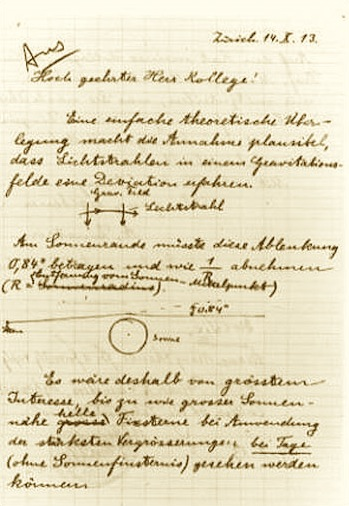Hale responded that it could not. The brightness of the sky near an uneclipsed sun is just too great.

In August 1914, there was a promising eclipse of the sun that would be visible from the Crimea. Einstein's colleague, the astronomer Erwin Freundlich, mounted an expedition to the Crimea to observe and photograph the eclipse.

Unfortunately for Freundlich, the First World War broke out. Since he was German, a citizen of an enemy nation, the Russians interned him and confiscated his equipment.

Fortunately for Einstein, no measurement was taken. Einstein's theory of 1914 was not yet the complete general theory of relativity. In his earlier theory, there was no curvature of ordinary space in the vicinity of the sun. As a result, as we saw in another chapter, his theory predicted the same deflection as Newtonian gravitation theory (assuming light consists of massive corpuscles). It was half the deflection predicted by the final theory. Had the test been carried out successfully, it would have produced a result that contradicted Einstein's earlier theory.

### Gravitational Slowing of Light

In 1907, Einstein had also concluded that the speed of light, and not just its direction, would be affected by the gravitational field. The effect was closely connected with the gravitational slowing of clocks and is almost entirely a consequence of the relativity of simultaneity. One can see how it comes about with a similar set of spacetime diagrams. The clocks A, A', B and B' all accelerate uniformly in a Minkowski spacetime and in a way that ensures that the distance from A to A' remains the same as from B to B'. A light signal propagates from A to A' and a second light signal propagates from B to B'.

The figure shows the hypersurfaces of simultaneity of an inertial observer. Of course the inertial observer will judge the two light signals to propagate at the same speed. That is just familiar special relativity.

We notice also that, initially, the four clocks A, A', B, B' run in synchrony according to the judgments of simultaneity of the inertial observer. Hence using the readings of these clocks directly, we will infer that the two light signals propagate at the same speed. In more detail, we note that the distance from A to A' equals the distance from B to B'; and each light signal takes the same time to traverse the distance. Both light signals leave when the local clocks read 0 and arrive when the local clocks read 3. Hence using these local clock readings, we infer that the two light signals travel at the same speed.Now consider how these processes are judged by an observer who accelerates with the clocks. All that changes in the analysis that follows is that we use different judgments of simultaneity. That leads to the judgment of differing speeds for the propagation of light.

Let us take the observer who accelerates with clock B. That observer's hypersurfaces of simultaneity will tilt more and more as clock B gains speed from the acceleration. This was the effect that led observer B to judge that the A clock was running slower than the B clock. This same tilting will lead observer B to judge that the AA' light signal propagates at roughly half the speed of the BB' light signal. Both signals traverse the same distance. However the the AA' signal leaves A when the B clock reads 0 and arrives at A' then the B clock reads 4. The BB' signal leaves B when the B clock reads 0 and arrives at B' when the B clock reads a little over 2.Recall that the judgments of simultaneity of accelerating observers who move with the clocks agree, since they agree on the hypersurfaces of simultaneity. So we can choose any one of the accelerating observers and get the same outcome. Each of the accelerating observers will judge the transit time for BB' to be roughly half that of AA'. They will agree that light propagates slower on the left side of the figure, that is, deeper in the created field.

Applying the principle of equivalence, we now conclude that the same slowing manifests in a gravitational field. A light signal deeper in the gravitational field at A propagates slower than a light signal higher in the gravitational field at B.

The conclusion that gravity slows the speed of light caused Einstein some trouble with unkind contemporary critics. Einstein had first based his theory of 1905 of the striking idea of the constancy of the speed of light, but he now seemed to be retracting it.

By 1912, Einstein had developed all these ideas into a fairly complete theory of static gravitational fields, that is gravitational fields that do not vary with time and admit well defined spaces. The most striking characteristic of the theory was that the intensity of the gravitation field, the gravitational potential, was given by the speed of light. So as one moved to different parts of space, the intensity of the gravitational field would vary in concert with the changes in the speed of light. As late as 1912, some five years after Minkowski's work, Einstein was loath to use spacetime methods. While I have developed the clock slowing and light slowing effects using spacetime diagrams, Einstein did not do this. His method of analysis was algebraic. He represented the processes by equations in which speeds and times appeared as variables. He rarely if ever drew diagrams such as given above.

What Einstein now needed was a way to extend these results to the more general case of gravitational fields that vary with time. That, it turned out, required Einstein to move well beyond the mathematics he knew. Another thought experiment pointed the way.

### The Rotating Disk

If one has a circular disk at rest in some inertial reference system in special relativity, the geometry of its surface is Euclidean. It will be useful to spell out what that means in terms of the outcomes of measuring operations. If the disk is ten feet in diameter, then it means that we can lay 10 foot long rulers across a diameter. Euclidean geometry tells us that the circumference is π x 10 feet, which is about 31 feet. That means that we can traverse the full circumference of the disk by laying 31 rulers around the outer rim of the disk.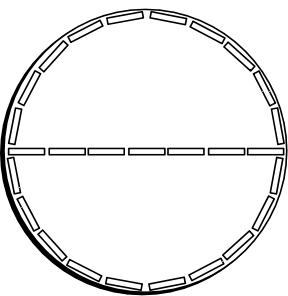What if we have a disk of the same diameter of 10 feet but in rapid uniform rotation with respect to the first disk? Things will go rather differently. Assume that this rotating disk is covered with foot long rulers that move with it. These rulers are just like the ones that were used to survey the non-rotating disk. (That means that an observer moving with the rod on the rotating disk would find it to be identical to one of the rulers used to survey the non-rotating disk.) What will be the outcome of surveying the geometry of this rotating disk with those rods? An observer who is not rotating with the disk would judge all these rulers to have shrunk in the direction of their motion. That means that, according to this new observer, the surveying of the disk would proceed differently. Ten rulers would still be needed to span the diameter of the disk. Since the motion of the disk is perpendicular to the rulers laid out along a diameter, the length of these rulers would be unaffected by the rotation. That is not so for the rulers laid along the circumference. They lie in the direction of rapid motion. As a result, they shorten. More rulers are needed to cover the full circumference of the disk. Note what was not said in this account. It did not say that we take the first disk and set it into rotation. The reason is that it is impossible in relativity theory to take a disk made out of stiff material and set it into rotation. If one were to try to do this, the disk would contract in the circumferential direction but not in the radial direction. As a result, a disk made of stiff material would break apart. If we want a rotating disk made of stiff material, we need to create it already rotating. Once, in a letter on the subject, Einstein remarked that a way to get a disk of stiff material into rotation is first to melt it, set the molten material into rotation and then allow it harden. The rotating disk problem has created a rather large and fruitless literature that suggests some sort of paradox is at hand. Most of it derives from a failure to recognize that a stiff disk cannot be set into uniform rotation without destroying it. Another little trap to avoid: While we have used the judgments of an observer not on the disk to infer the outcome of the surveying operations on the disk, the outcomes of those operations are independent of the observer's state of motion. Either a diameter can be covered with ten rods laid on its surface or it cannot; either the circumference can be spanned by 31 rulers or it cannot. Once one observer has found which is the case, we know the result for all observers.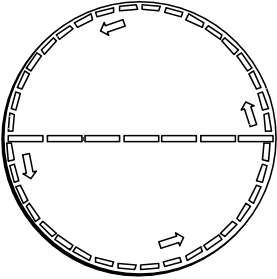Thus we measure the circumference of the rotating disk to be greater than 31 feet, the Euclidean value. In other words, we find that the geometry of the disk is not Euclidean. The circumference of the disk is more than the Euclidean value of π times its diameter.

The significance of this thought experiment was great for Einstein. Through his principle of equivalence, Einstein had found that linear acceleration produces a gravitational field. Now he found that another sort of acceleration, rotation, produces geometry that is not Euclidean.

### Assembling the Pieces: The "Entwurf" Paper of 1913

Einstein had all this in place by the summer of 1912. He knew that gravitation could bend light and slow clocks. He expected that the final theory would somehow involve accelerations in a new way and that such accelerations came with a breakdown of Euclidean geometry. He also knew that the natural arena in which to conduct relativity theory is Minkowski's spacetime.

 Gravity slows clocks and bends light. Uniform, unidirectional acceleration produces a homogeneous gravitational field. Rotational acceleration leads to spaces with non-Euclidean Geometries. Spacetime is the appropriate arena for relativity.To us, the final step does not seem like such a great leap. Assemble the pieces and infer that gravitation is a curvature of spacetime! All that is needed is a nice mathematical clothing to dress this idea. For Einstein in 1912 it was far from easy. He first needed the assistance of his mathematician friend Marcel Grossmann to find his way in the new and difficult mathematics the theory required.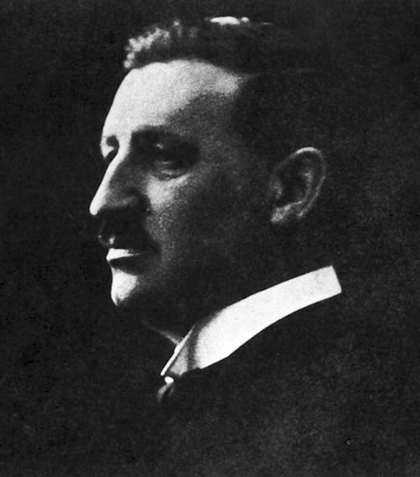Marcel Grossmann

Grossmann visited the library and found a review article on the  "absolute differential calculus" of Ricci and Levi-Civita from 1901. (We now call it "tensor calculus.") It enabled the equations of a physical theory to be written in any coordinate system. This, in Einstein's view, was just the mathematical instrument needed, for Einstein had associated an extension of the principle of relativity to acceleration with an expansion of the spacetime coordinate systems of a theory.

 The two collaborated on the paper shown opposite. It is called, in translation, "Sketch [Entwurf] of a Generalized Theory of Relativity and a Theory of Gravitation." They divided the work. Grossmann, the mathematician,  developed the complicated mathematics, mostly then unknown to physicists. This occupied the second part: "II. Mathematical Part." The physical reasoning in which the theory itself was developed was to be written by Einstein in the first part: "I. Physical Part." To a modern relativist, the paper is a little odd for the antiquated notation Einstein and Grossmann used. However most of the content is familiar. The spacetime of Minkowski is generalized to embrace gravitational phenomena by allowing for what we would now call curvature of the spacetime. The formalism of what we now call "tensor calculus" is developed and used to state the main results. The law of conservation of energy and momentum appears in a form that is equivalent to that of the modern textbooks.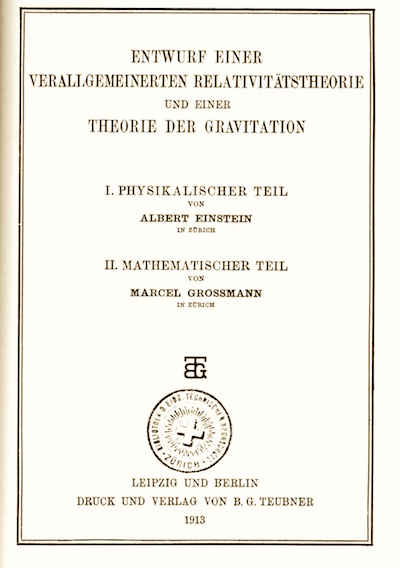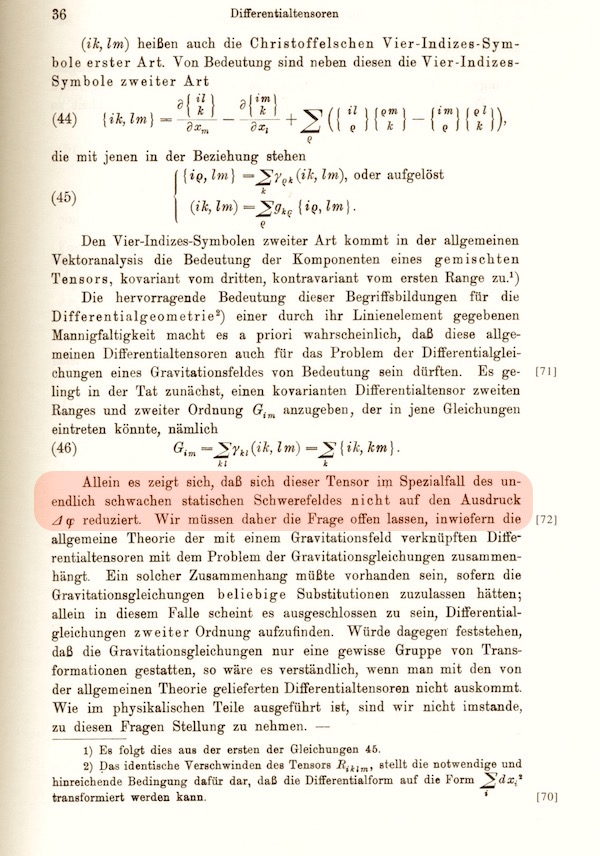Then comes the key question of the gravitational field equations. Here things started well, at least in Grossmann's mathematical part. From a mathematical perspective, the place to start was the Riemann curvature tensor. It is the quantity that measures the curvature of all the different sheets of spacetime. Grossmann introduced it on the page opposite as the the "Christoffel four-index-symbol of the first kind" (ik, lm). He then performed the mathematical operation of contraction on it to yield the expression Gim seen in the middle of the page. This quantity is almost the Einstein tensor. Grossmann is so close to the modern Einstein field equations that one could now scarcely imagine how the final steps could not be taken. But they are not taken. One condition the new equations must satisfy is that they must return Newtonian results for ordinary conditions. For Newton's theory works extraordinarily well for the weak, static gravitational fields of our solar system. The sentence highlighted in red says: "However it turns out the this tensor does not reduce to the [Newtonian expression] Δφ in the case of infinitely weak, static gravitational fields." That is, Grossmann declared the equations under consideration not to merge nicely back into Newton's theory in this essential case. With that declaration, the paper turns from the road that was leading directly to the Einstein field equations.
 With the catastrophic turn taken, Einstein took up the search for gravitational field equations in his physical part. He was guided by the few clues he had at hand: his earlier results of 1907-1912 on static gravitational fields, the requirement that the theory merge back into Newtonian theory and the requirement of conservation of energy and momentum. Einstein eventually found equations that he believed adequate. They are complicated and have been given no clear geometric meaning. Parts of them are spread over the page opposite. If they look complicated to you, don't fret. That also was the reaction of Einstein's contemporaries. One simple equation of Newton's theory had been replace by ten, non-linear, coupled equations of Einstein's theory.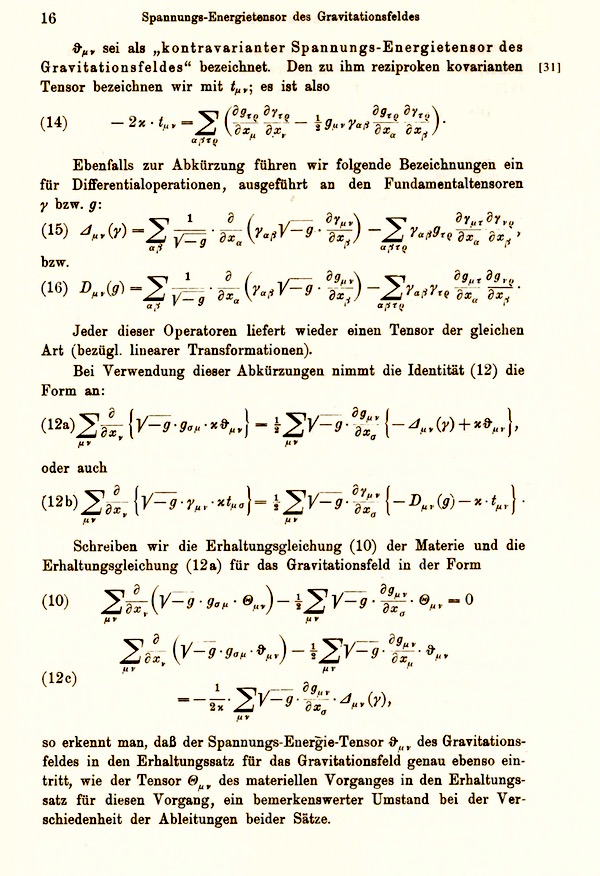### What Went Wrong?

Einstein took a series of bad turnings and ended up with the wrong gravitational field equations--not the celebrated Einstein equations that appear in all the modern textbooks. He needed over two years of painful work first to recognize that something had gone wrong and then to find the final equations.

The precise causes that brought about these wrong turnings remain a point of debate in the history of general relativity literature. We can identify two elements, however, that played a role in misleading Einstein.First, in 1912 and 1913, Einstein had recognized the need to employ a geometry of variable curvature in spacetime in his theory of gravity. However he was convinced that this curvature would not be manifested in the space-space slices of spacetime in certain simple cases. These were the cases of a static gravitational field and also of very weak gravitational fields. These two cases combined is just the circumstance identified by Grossmann as troublesome. Both of them are realized in the gravitational field of the sun. Einstein expected space around the sun to be exactly Euclidean. Alas, as we have seen, Einstein's final theory requires curvature in the space-space slices even in this simple case. That meant that Einstein and Grossmann could not accept the equations of the final theory for they would entail a curvature of space when Einstein believed there was none.

Second, Einstein used a different style of theorizing to the one largely used in these chapters. Here, we have used a geometrical approach, emphasizing the picturing of gravitational effects in geometric diagrams. Einstein, however, labeled events in spacetime with arbitrarily coordinate numbers and expressed all his results in terms of equations relating these coordinates. Einstein knew that this labeling of spacetime events with coordinates was purely arbitrary and that all his results had to be independent of the particular coordinate system used. However knowing this in the abstract and carrying through the demand in all details are two different things. Einstein's "Entwurf" field equations applied only to specialized sets of coordinate systems. He called them "adapted." By his own later admission, Einstein had found it hard to purge his coordinate systems of independent reality.

One of the low points in his struggle with coordinate systems came when Einstein used an ingenious argument--the "hole argument"--to show that gravitational field equations like the ones of his final theory are inadmissible on physical grounds. While the hole argument did not warrant that conclusion, it has been rehabilitated in recent work in philosophy of space of time, where it now lives a good life.

For an elaboration, see the later chapter "The Philosophical Significance of General Relativity: The Hole Argument." (See also, "The Hole Argument." Stanford Encyclopedia of Philosophy.)

### The Zurich Notebook

 The time of Einstein's collaboration with Grossmann that led up to the "Entwurf" paper is the decisive period for the discovery of general relativity. For it is when Einstein takes the hunches and results of his earlier speculations and molds them into the theoretical framework of general relativity. It would be wonderful if we could look over Einstein's shoulder during this period and watch him work. We would see the creation of general relativity. Of course, 1912 and 1913 are now in the past. We can do something almost as good. The private calculations Einstein made during this time have survived. The pages of the Zurich Notebook enable us to watch the steps Einstein took, from the earliest moment when he connected gravitation with spacetime curvature, through the evaluation and rejection of his later field equations, on to the equations of his "Entwurf" theory.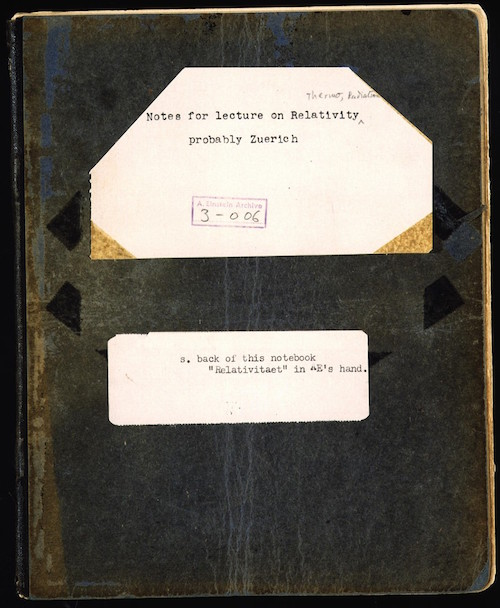For a more detailed glimpse into Einstein's notebook, see "A Peek into Einstein's Zurich Notebook." on my Goodies page. Here's one page on which Einstein writes down the Riemann curvature tensor for the first time and finds it hard to see how it can be used in his gravitational field equations.The notebook has many wonderful insights into the detailed steps of Einstein's work; and there are still pages whose content eludes us. Each is a little puzzle for us solve. One remarkable page that defeated me was solved by Tilman Sauer. It shows Einstein playing with  the key ideas of his new theory in a way that does not persist into later publication.It will not be at all obvious what Einstein is computing on the right hand side of this page. At least it was not to us for a long time. So let me tell you what Einstein computes.

 In general relativity, a body in free fall traces out a geodesic of the spacetime. That is, it follows a trajectory of extremal proper time. There is a result which looks unnervingly close to this in ordinary classical physics. Take a curved surface. Have body that can move freely in it, except that it is not allowed to leave the surface. An example is shown opposite: a particle is captured by the curved surface of a cup. While the body skims over the surface of the cup, what curve is traced out in the surface of the cup? It is a geodesic of cup's surface! That is, it traces a curve of shortest spatial length. That fact is what Einstein deduces on this page.The result is so close to the central idea of Einstein's general theory of relativity that one has to look closely to see how the two differ:

 Classical physics General relativity A mass moves freely in space, except that it is constrained to a two-dimensional surface in the three-dimensional space. A mass moves freely in spacetime. That is, it is in free fall, so that gravity acts on it through the curvature of spacetime. Its spatial trajectory is a geodesic of the two-dimensional surface. That is, it traces of curve of shortest length in the surface. Its spacetime trajectory is a geodesic of the spacetime. That is, it traces a curve of extremal spacetime interval in spacetime.

### November 1915: The Race to the Finish

With the publication of the "Entwurf" paper in mid 1913, Einstein felt that the major work on the theory was done. Only the details needed to be clarified. The feeling did not last. As the months and years passed. Einstein worked harder and harder to convince himself that all was well with a theory that was misshapen in its fundamental equations.

In the summer and fall of 1915, the clues that his old theory was wrong mounted. He knew that his theory of 1913 did not accommodate the anomalous motion of Mercury. He then found that it did not extend the relativity of motion to rotation. Finally, he found that an improved and much more sophisticated development of the theory of late 1914 did not demonstrate its uniqueness, as he then believed.

In mounting despair and desperation to save his theory, he returned to the thinking of 1912 and 1913. He was mistaken, he saw, to restrict his theory only to the special "adapted" coordinate systems of the "Entwurf" theory. He needed a theory that would work in as many coordinate systems as possible. Ideally it would work in all coordinate systems. That is, it would be "generally covariant."

What followed was the most exhausting and exhilarating month of Einstein's life. He sent the Prussian academy bulletins on his reformulated theory, one per week, each correcting errors and incompleteness of the previous bulletins. What no doubt lent special urgency to this extraordinary behavior was the fact that David Hilbert, the greatest mathematician of the era, had also become interested in the theory and had started the project of rewriting the gravitational field equations of Einstein's old "Entwurf" theory in a mathematically more elegant formulation.

The first communication was sent on November 4 and was written as it if was the last.It was not. The following week, November 11, Einstein sent a correction.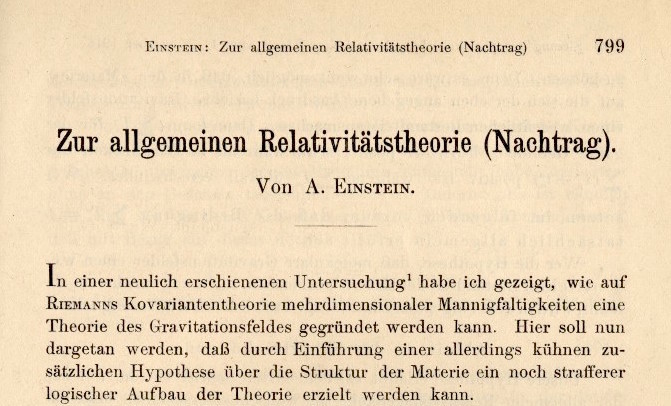And the following week, November 18, a jubilant Einstein communicated the remarkable news that his newly formed theory accounted for the anomalous motion of Mercury. ("Explanation of the perihelion motion of Mercury by the general theory of relativity.")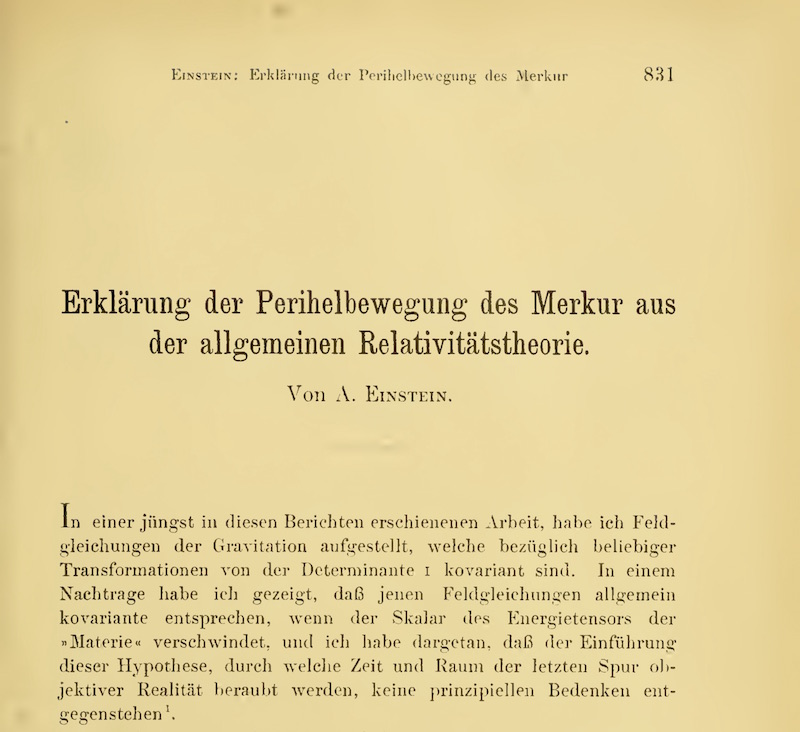The field equations of the theory were still not quite right. It was only in the fourth communication of November 25 that the final equations appeared.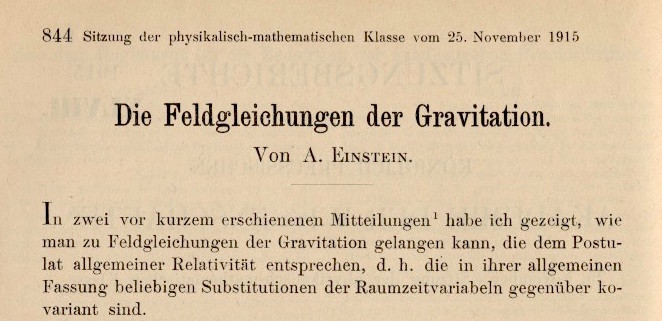Here are those equations as he published them for the first time on 25 November 1915.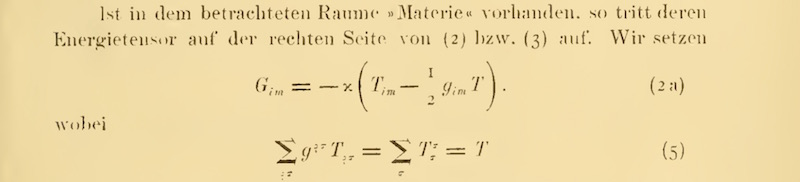Here he writes them later in the simple case of a matter free spacetime: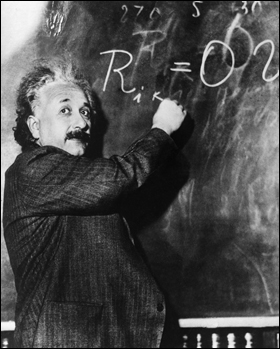## What You Should Know

• What first led Einstein to work on what became his general theory of relativity.
• The principle of equivalence
• How Einstein used it to infer the properties of gravitational fields.
• The relativity of inertia.
• Einstein's transition to the mathematics of spacetime curvature.

Copyright John D. Norton. February 2001; January 2, 2007, February 15, August 23, October 16, 27, 2008; February 5, 19, 2010. Minor edits February 26, 2013. More December 29, 2015. January 15, February 27,  2017. LInks added November  16, 2019. February 4, 2022.